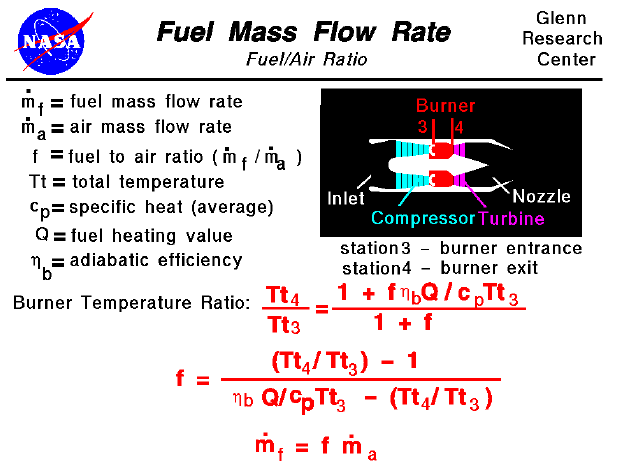A text only version of this slide is available which gives all of the flow equations. The interactive Java applet EngineSim is also available. This program solves these equations and displays the thrust and fuel flow values for a variety of turbine engines.

The propulsion system of an aircraft must perform two important roles:

• During cruise, the engine must provide enough thrust, to balance the aircraft drag while using as little fuel as possible.
• During takeoff and maneuvers, the engine must provide excess thrust to accelerate the aircraft.
Most modern passenger and military aircraft are powered by gas turbine engines, which are also called jet engines. There are several different types of jet engines, but all jet engines have some parts in common. All jet engines have a combustor or burner in which the air and fuel are mixed and burned. The thermodynamics of the burner play a large role in both the generation of thrust and in the determination of the fuel flow rate for the engine.

On this page we show the thermodynamic equations which relate the the temperature ratio in the burner to the fuel mass flow rate. The fuel mass flow rate (mdot f) is given in units of mass per time (kg/sec). The fuel mass flow rate is related to the total engine air flow rate (mdot a) by the fuel to air ratio (f). The energy equation for the burner can be solved for the temperature ratio across the burner:

Tt4 / Tt3 = (1 + f * nb * Q / (cp * Tt3)) / (1 + f)

where Tt4 is the burner exit temperature, Tt3 is the burner entrance temperature, Q is the fuel heating value, cp is the specific heat coefficient of air at constant pressure and nb is an efficiency factor to account for losses in the burner.

Using the temperature equation, and a little algebra, it is possible to solve for the fuel to air ratio (f). From the fuel to air ratio, we can obtain the fuel mass flow rate by a simple multiplication.

f = [(Tt4 / Tt3) - 1] / [(nb * Q) / (cp * Tt3) - Tt4 / Tt3]

mdot f = f * mdot a

The engine air flow rate is normally set by conditions in the nozzle. We can use the value of fuel to air ratio to determine the engine's specific fuel consumption. As explained in another section of the Beginner's Guide to Aerodynamics, the specific fuel consumption and the aircraft fuel load determine the maximum flight time and the maximum range of an aircraft.

It would appear that we can make the temperature ratio (and resulting thrust) as large as we want by just increasing the fuel flow rate (and the fuel/air ratio). However, the details of the combustion process sets some limits on values of the fuel/air ratio. And in engine operation, there is a maximum burner exit temperature (Tt4) which is determined by materials limits (http://www.ueet.nasa.gov/parts.htm). If we try to run the engine hotter than this maximum temperature, the burner and the turbine will be damaged. You can now use EngineSim to study the effects of different materials on engine operation.Guided Tours